Would you rather have \$24,000 today, or \$25,000 one year from today?

## Present Value Definition

Simply defined, present value takes a value that you will receive in the future, and tells you how much it would be worth if you had it today.

You may not think that's a very useful tool; I mean, how often does someone offer you the choice between money today and money tomorrow? However, we use present value calculations every day. Want to make sure you have enough money to put your kid through college by the time he's 18? Present value can help you calculate how much money you need to invest today to make that goal a reality.

It starts with the assumption that any dollar you receive today will be invested (and can start earning interest) immediately. Based on that, a dollar you receive today will be worth more than a dollar you receive in the future.

To calculate the present value of money you will collect later, you must also know:

• The amount you will receive in the future (also known as the future value)

• How much time will pass before you will receive the money

• The interest rate you could be receiving if that money were invested now

• How that interest would be calculated

If we're talking about one period, like the one year in our example at the top, the formula for present value is simple: divide the future value (amount money will be worth in the future) by one plus the applicable interest rate.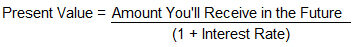For example, if the amount you will receive one year from now is \$25,000 and you know your savings account will pay you 7% a year, the present value is \$23,364, as shown in the calculation below: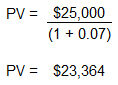Getting back to the original question, if you assume a 7% interest rate, collecting \$24,000 today is the superior choice since the present value of \$25,000 received one year from now is less at \$23,364.

## Simple versus Compound Interest

In this first example, we used simple interest. Simple interest is the most basic way to calculate interest; you simply apply the interest rate against the original investment amount. So, the annual interest payment on a \$100 loan with a simple interest rate of 5% a year would be \$5.

However, most investments pay compound interest, and this dramatically impacts the calculation. With compound interest, the principal amount and the interest you receive both earn additional interest. Your balance escalates as your holding period increases, resulting in a much larger future value and a smaller present value.

Let's look at an example.

To calculate the present value of \$25,000 when the interest compounds daily over the next year, first we need to know what our daily rate is. We do this by dividing the annual rate (0.07) by 365 to get 0.000192. This new rate is called our 'periodic rate' because it's the interest rate over the period we're interested in -- in this case, days.

Now we have to use a slightly more complicated formula: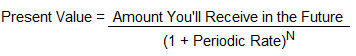where the periodic rate is our daily rate (0.000192) and N is the number of periods. Because we're compounding interest every day, N = 365 days.

Using the data from our example, we use a financial calculator to calculate present value as: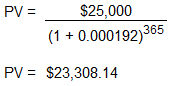## Calculating Present Value in Multiple Periods

Present value is especially useful when you use it to calculate your earnings over multiple periods. The formula for present value in this scenario is: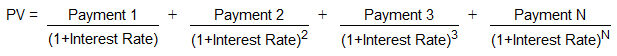Where N measures the number of periods.

Let's look at an example.

Suppose you hold an investment that will pay \$10,000 each year over the next three years and the interest rate is 7%. The present value of that investment is \$26,243.10 as shown below: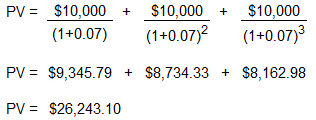## How Present Value Impacts Your Investments

As an investment tool, the concept of present value is useful because it allows investors to compare two investments with different payback terms and determine which is better.

For example, suppose you are given a choice between receiving \$12,000 in one year or \$1,000 per month for 12 months. The two investments may look identical -- after all, they both return \$12,000, but the concept of present value shows one option is clearly superior to the other.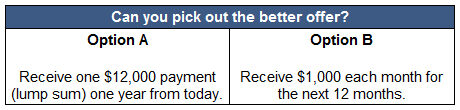Assuming a 7% interest rate, we calculate the present value of the two options as follows: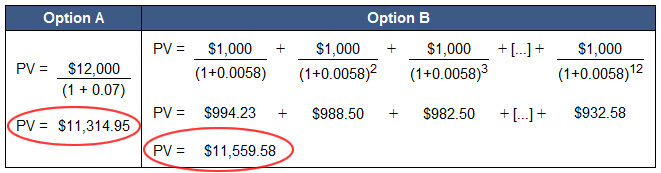In Option A, we use the formula as we first introduced it to you. Pretty straightforward.

In Option B, we had to add in a few additional twists. First, we are evaluating a series of \$1,000 payments over 12 months. We begin with the annual interest rate of 7%, but the present value of each payment is calculated individually, so we need to divide the annual rate by 12 to get the monthly rate (this is known as the periodic rate). In this example, the periodic interest rate is 7%/12, or 0.58%.

The sum total of the 12 individual present value calculations is \$11,559.58. Comparing the two options shows the monthly payments have a higher present value today than the lump sum.

Knowing the formulas is practical, but present value can also be calculated using the tables provided in many finance and/or accounting textbooks or by using the present value function available in Excel spreadsheets. In fact, we've developed a tutorial that will teach you to calculate present value with Excel or, if you prefer, calculate present value with a financial calculator.

## Drawbacks of Present Value

Just like other valuation methods, there are limits to what present value can do. A major drawback is that the present value formula is inflexible. For example, if payments become riskier in later periods or if more payment options become available, these events can't be built into the present value model.

Present value is also highly sensitive to even small changes in the interest rate or payment stream. For example, if you are calculating the present value of an ongoing business, the last payment is used to estimate payments for all future periods. If the estimate is even a little too high, the end result is a wildly overinflated present value for the business.

Even so, present value is one of the most important concepts in finance and investing. If you want to learn more, it may be beneficial to look at present value's cousin, future value. In our featured article, The Greatest Mathematical Discovery of All Time, we explain why compound interest is so powerful and we walk you through the steps to calculate the future value of your investments.